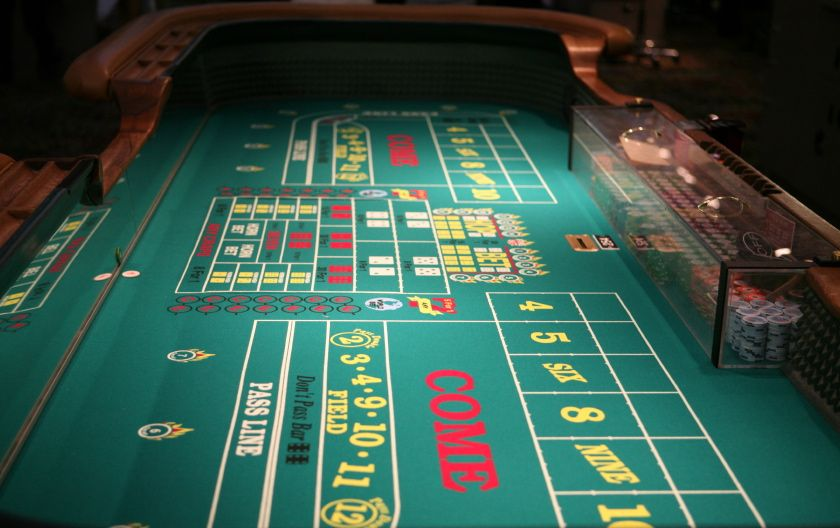# Odds in the Game of Craps

April 1, 2020

## Using Probability to Calculate the Odds in the Game of Craps

Craps is a dice game that never fails to catch the eyes of the visitors in a casino. A fun-filled exciting themed dice dame can go both good and worse for you if you do not play your cards right. Although the basic strategy for the game is based on luck and chance, probability also plays a vital role in his game. Of course, you cannot change the outcomes of the dice, but you can strategize the wins and losses in such a way that you don’t go bankrupt at the end of the game.

#### Craps probabilities

First, let us understand how the probability of craps works. Consider two dices, which are generally of six sides, such that when you roll the two dice, you have 36 different outcomes. Consider that the most frequent outcome is seven which covers 6 of the 36 outcomes. On the contrary, 2 and 12 are the least outcomes, and they cover only one of the 36 different possible outcomes. Hence it is 6+6 and 1+1.

In a pass line bet, which is one of the easiest and offers the lowest house edge, you have to check for the probability of getting 7 or 11 as the outcome. From our above calculation, we know that it is 6 and 2. Hence your chances of winning for every particular roll will be 6+2 =8/36.

On the contrary, the risk of losing the game is when the outcomes are 2,3, and 12, which sums up to 4/26 of the possible outcomes. Therefore, you have twice the chance of winning the come-out roll than losing it. However, as the game continues, these odds are staged differently and hence show drastic changes.#### Example

Consider rolling a six-sided die whose outcomes can be one 1,2,3,4,5, and 6. This means every time an extra die is added. The outcome is multiplied by 6. So, if you roll two dice, then the possible outcome is 36 (6X6).

If you want the target outcome as 11, then you can achieve it either by rolling 5-6 or 6-5. Hence, here your target outcome is two, and the total outcome is 36.

Therefore probability of 11 = 2/36= 1/18

This means that you have 1 in 18 chances that you get the desired outcome of 5-6 or 6-5.

#### The probability of finding the odds

You can use this basic strategy and formula to find out your odds of winning. Your first roll is called the come-out roll, which can have one of the three outcomes

• 7 or 11: Automatically wins
• 2,3, or 12: Automatically lose
• 4,5,6,8,9,10: Point for the next round

Hence, depending on the possible outcome, you wish to achieve and the total number of outcomes, you can calculate your odds of winning or losing the game. After your come-out roll, they don’t work come out also have a better chance of winning if you lose. While calculating the probability, it is essential to note the house edge, which is the least or pass line bets.

Odds in the Game of Craps